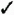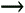# Section 44. About Calc FormulasBEFORE YOU BEGINSEE ALSO

45 Copy and Move Formulas

Without formulas , Calc would be little more than a simple row-and-column-based word processor. When you use formulas, however, Calc becomes an extremely powerful timesaving, planning, budgeting, and general-purpose financial tool.KEY TERM

Formulas Equations composed of numeric values and often cell addresses and range names that produce a mathematical result.

On a calculator, you typically type a formula and then press the equal sign to see the result. In contrast, all Calc formulas begin with an equal sign. For example, the following is a formula:

` =4*2-3 `

The asterisk is an operator that denotes the times sign (multiplication). This formula requests that Calc compute the value of 4 multiplied by 2 minus 3 to get the result. When you type a formula and press Enter or move to another cell, Calc displays the result and not the formula on the worksheet.

When you enter =4*2-3 in a cell, the answer 5 appears in the cell when you move away from the cell. You can see the formula in the Input line atop the sheet if you click the cell again to make it active. When entering a formula, as soon as you press the equal sign, Calc shows your formula in the Input line area as well as in the active cell. If you click the Input line first and then finish your formula there, the formula appears in the Input line as well as in the active cell. By typing the formula in the Input line, you can press the left- and right-arrow keys to move the cell pointer left and right within the formula to edit it.

Table 6.3 lists the primary math operators you can use in your worksheet formulas. Notice that all the sample formulas begin with the equal sign.

##### Table 6.3. The Primary Math Operators Specify Math Calculations

Operator

Example

Description

^

=7 ^ 3

Raises 7 to the power of 3 (called exponentiation )

/

=4 / 2

Divides 4 by 2

*

=3 * 4 * 5

Multiplies 3 by 4 by 5

+

=5 + 5

=5 - 5

Subtracts 5 from 5

Calc displays a formula's result in the cell.You can combine any and all the operators in a formula. When combining operators, Calc follows the traditional computer (and algebraic) operator hierarchy model . Therefore, Calc first computes exponentiation if you raise any value to another power. Calc then calculates all multiplication and division in a left-to-right order (the first one to appear computes first) before addition and subtraction (also in left-to-right order).KEY TERM

Operator hierarchy model A predefined order of operators when equations are being calculated.

The following formula returns a result of 14 because Calc first calculates the exponentiation of 2 raised to the third power and then divides the answer (8) by 4, multiplies the result (2) by 2, and finally subtracts the result (4) from 18. Even though the subtraction appears first, the operator hierarchy forces the subtraction to wait until last to compute.

` =18 - 2 ^ 3 / 4 * 2 `

If you want to override the operator hierarchy, put parentheses around the parts you want Calc to compute first. The following formula returns a different result from the previous one, for example, despite the same values and operators used:

` =(18 - 2) ^ 3 / 4 * 2 `

Instead of 14 , this formula returns 2,048 ! The subtraction produces 16, which is then raised to the third power (producing 4,096) before dividing by 4 and multiplying the result by 2 to get 2,048.NOTE

To add three cells together, you could type the following in another cell:

`  =D3+K10+M7  `

Calc adds the values in D3, K10 , and M7 and shows the result in place of the formula. The cells D3, K10 , and M7 can also contain formulas that reference other cells.Sams Teach Yourself OpenOffice.org 2, Firefox and Thunderbird for Windows All in One
ISBN: 0672328089
EAN: 2147483647
Year: 2005
Pages: 232
Authors: Greg Perry